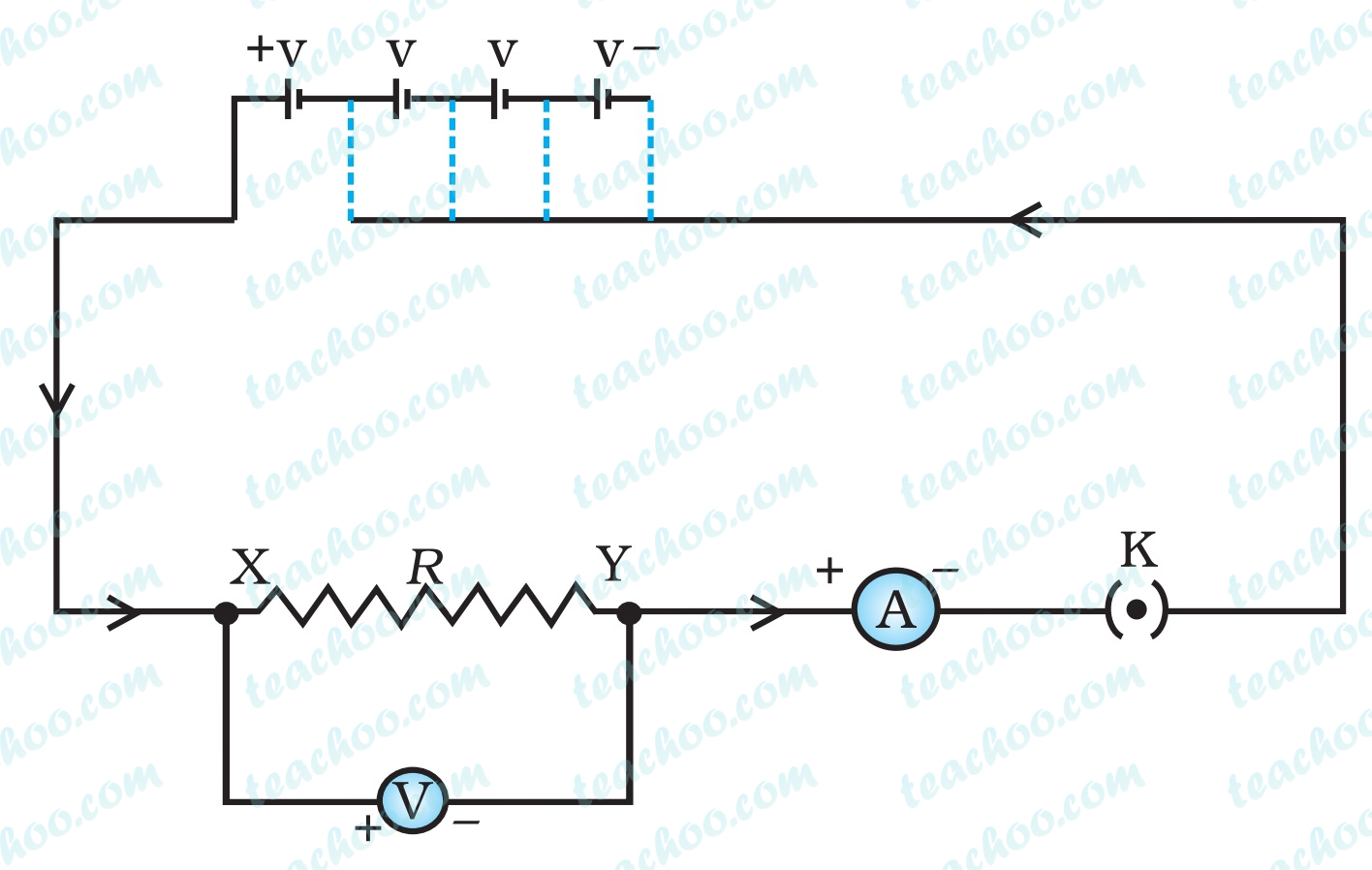Questions from Inside the chapter

Class 10
Chapter 12 Class 10 - Electricity

## (b) Which material is the best conductor?(a)

Resistivity of iron = 10 × 10 -8

Resistivity of mercury = 94 × 10 -8

Resistance is directly proportional to the resistivity.

Higher the resistivity, the higher the resistance.

Hence, resistance of mercury is more than resistance of iron.

Since iron would provide lower resistance to the flow of current, iron is a better conductor.

(b) The substance which has the lowest resistivity is the best conductor.

Silver has the lowest resistivity.

Hence, silver is the best conductor.

Learn in your speed, with individual attention - Teachoo Maths 1-on-1 Class